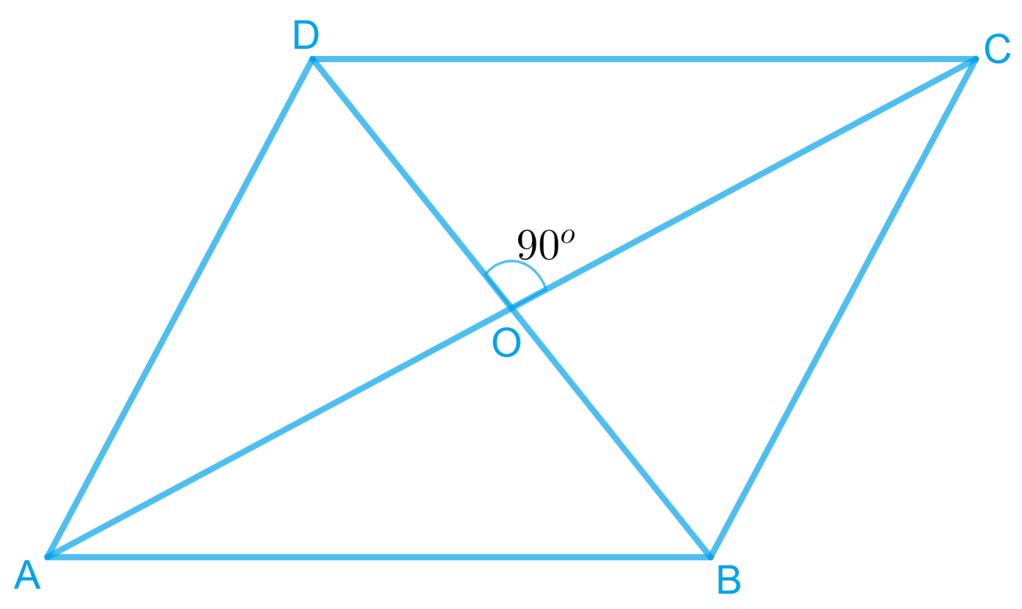# Ex.8.1 Q3 Quadrilaterals Solution - NCERT Maths Class 9

Go back to  'Ex.8.1'

## Question

Show that if the diagonals of a quadrilateral bisect each other at right angles, then it is a rhombus.

Video Solution
Ex 8.1 | Question 3

## Text Solution

What is known?

The diagonals of a quadrilateral bisect each other at right angles.

What is unknown?

How we can show that it is a rhombus.

Reasoning:

To show that given quadrilateral is a rhombus, we have to show it is a parallelogram and all the sides are equal.

Steps:Let  be a quadrilateral, whose diagonals  and  bisect each other at right angle i.e

$$\mathrm{OA}\!=\!\mathrm{OC}, \mathrm{OB}\!=\!\mathrm{OD},$$ and

$$\angle \mathrm{AOB}\!=\!\angle \mathrm{BOC}\!=\!\angle \mathrm{COD}\!=\!\angle \mathrm{AOD}\!=\!90^{\circ}$$

To prove $$ABCD$$ a rhombus,

We have to prove $$ABCD$$ is a parallelogram and all the sides of $$ABCD$$ are equal.

In $$\Delta A O D \text { and } \Delta C O D,$$

\begin{align}O A&=O C\\text { Diagonals }&\text{bisect each other )} \\\\ \angle A O D&=\angle C O D \quad \text { (Given) } \\ O D&=O D \quad \text { (Common) } \\ ∴ \Delta A O D &\cong \Delta C O D \\ \text { (By SAS}&\text{ congruence rule) } \\\\ \therefore A D&=C D\quad\dots\text{(1)}\end{align} Similarly, it can be proved that \begin{align}A D=A B \text { and } C D=B C\quad\dots\text{(2)}\end{align} From Equations (1) and (2), \begin{align}A B=B C=C D=A D\end{align} Since opposite sides of quadrilateral \(ABCD are equal, it can be said that $$ABCD$$ is a parallelogram. Since all sides of a parallelogram $$ABCD$$ are equal, it can be said that $$ABCD$$ is a rhombus.

Video Solution